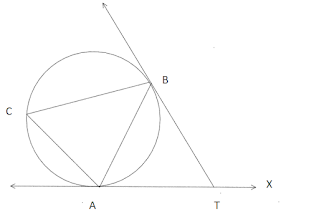# Myanmar Matriculation 2019 Inland

$\def\mathrm{}\def\frac{\dfrac}$

2019

2019
MATRICULATION EXAMINATION

DEPARTMENT OF MYANMAR EXAMINATION

 MATHEMATICS Time Allowed : (3) Hours

SECTION A
 1 (a) Functions $\mathrm{f}$ and $\mathrm{g}$ are defined by $\mathrm{f}(\mathrm{x})=\mathrm{x}+1$, and $\mathrm{g}(\mathrm{x})=2 \mathrm{x}^{2}-\mathrm{x}+3$. Find the values of $\mathrm{x}$ which satisfy the equation $(\mathrm{f} \circ \mathrm{g})(\mathrm{x})=4 \mathrm{x}+1$. (3 marks) Click for Solution (b) The expression $2 x^{2}+5 x-3$ leaves a remainder of $2 p^{2}-3 p$ when divided by $2 x-p$. Find the values of $\mathrm{p}$. (3 marks) 2 (a) Find and simplify the coefficient of $\mathrm{x}^{7}$ in the expansion of $\left(\mathrm{x}^{2}+\frac{2}{\mathrm{x}}\right)^{8}, \mathrm{x} \neq 0 .$ (3 marks) (b) Find the sum of all even numbers between 69 and 149 . (3 marks) Click for Solution 3 (a) The matrices $\mathrm{A}=\left(\begin{array}{ll}2 & 0 \\ 0 & 5\end{array}\right)$ and $\left(\begin{array}{ll}\mathrm{x} & \mathrm{y} \\ 0 & \mathrm{z}\end{array}\right)$ are such that $\mathrm{AB}=\mathrm{A}+\mathrm{B}$. Find the values of $\mathrm{x}, \mathrm{y}$ and $\mathrm{z}$ (b) A die is thrown. If the probability of getting a number not less than $\mathrm{x}$ is $\frac{2}{3}$, find $\mathrm{x}$. (3 marks) Click for Solution 4 (a) AT and BT are tangents to the circle $A B C$ at $\mathrm{A}$ and $\mathrm{B}$. Prove that $\angle \mathrm{BTX}=2 \angle \mathrm{ACB}$. (3 marks)(b) The coordinates of $\mathrm{A}, \mathrm{B}$ and $\mathrm{C}$ are $(1,0),(4,2)$ and $(5,4)$ respectively. Use vector method to determine the coordinates of $\mathrm{D}$ if $\mathrm{ABCD}$ is a parallelogram. (3 marks) Click for Solution 5 (a) Solve the equation $2 \cos x \sin x=\sin x$ for $0^{\circ} \leq x \leq 360^{\circ}$. (3. marks) (b) Differentiate $\mathrm{x}^{3}+2 \mathrm{x}$ with respect to $\mathrm{x}$ from the first principle. (3 marks) Click for Solution

#### SECTION B

 6 (a) The functions $f$ and $g$ are defined by $f(x)=2 x-1$ and $g(x)=4 x+3$. Find $(g \circ f)(x)$ and $g^{-1}(x)$ in simplified form. Show also that $(g \circ f)^{-1}(x)=\left(f^{-1} \circ g^{-1}\right)(x)$. (5 marks) (b) The expression $a x^{3}-x^{2}+b x-1$ leaves the remainders of $-33$ and 77 when divided by $\mathrm{x}+2$ and $\mathrm{x}-3$ respectively. Find the values of $\mathrm{a}$ and $\mathrm{b}$, and the remainder when divided by $\mathrm{x}-2$ (5 marks) Click for Solution 7 (a) A binary operation $\odot$ on R is defined by $x\odot y = (3y−x)^2 −8y^2.$ Show that the binary operation is commutative. Find the possible values of $k$ such that $2 \odot \mathrm{k}=-31.(5 \mathrm{marks}$ (b) If the coefficients of $\mathrm{x}^{\mathrm{r}}$ and $\mathrm{x}^{\mathrm{r}+2}$ in the expansion of $(1+\mathrm{x})^{2 \mathrm{~m}}$ are equal, show that $r=n-1 .$ $(5$ marks) Click for Solution 8 (a) Find the solution set in $\mathrm{R}$ of the inequation $\mathrm{x}^{2}-3 \mathrm{x}+2 \leq 0$ by algebraic method and illastrate it on the number line. $\quad$ (5 marks) (b) Find the sum of the first 12 terms of the A.P. 44,$40 ; 36, \ldots \ldots .$ Find also the sum of the terms between the $12^{\text {th }}$ term and the $26^{\text {th }}$ term of that A.P. (5 marks) Click for Solution 9 (a) The sum of the first n terms of a certain sequence is given by $\mathrm{S}_{\mathrm{n}}=2^{\mathrm{n}}-1$. Find the first: 3 terms of the sequence and express the $\mathrm{n}^{\text {th }}$ term in terms of n. $\quad$ (5 marks) (b) Using the definition of inverse matrix, find the inverse of the matrix $\left(\begin{array}{ll}3 & 1 \\ 2 & 1\end{array}\right) .(5$ marks $)$ Click for Solution 10 (a) Find the inverse of matrix $\left(\begin{array}{ll}5 & 6 \\ 7 & 8\end{array}\right)$. Use it to determine the coordinates of the point of intersection of the lines $5 x+6 y=7$ and $8 y+7 x=10 . \quad$ (5 marks) (b) Construct the table of outcomes for rolling two die. Find the probability of an outcome in which the score on the first die is less than that on the second die. Find also the probability that the score on first die is prime and the score on the second is even. ( 5 marks) Click for Solution

#### SECTION (C)

 11 (a) PT is a tangent and PQR is a secant to a circle. A circle with $\mathrm{T}$ as centre and radius TQ. meets QR again at S. Prove that $\angle \mathrm{RTS}=\angle \mathrm{RPT} . \quad$ (5 marks) Click for Solution (b) In the diagram, $\mathrm{P}$ is the point on $\mathrm{AC}$ such that $\mathrm{AP}=3 \mathrm{PC}, \mathrm{R}$ is the point on BP such that $\mathrm{BR}=2 \mathrm{RP}$ and $\mathrm{QR} / / \mathrm{AC}$. Given that $\alpha(\triangle \mathrm{APA})=36 \mathrm{~cm}^{2}$, calculate $\alpha(\Delta \mathrm{BPC})$ and $\alpha(\Delta \mathrm{BRQ}), \quad \mathrm{Q} \quad \mathrm{A}$ (5 marks)12 (a) Prove that the quadrilateral formed by producing the bisectors of the interior angles of any quadrilateral is cyclic. ( 5 marks) (b) If $\alpha+\beta+\gamma=180^{\circ}$, prove that $\tan \frac{\alpha}{2} \tan \frac{\beta}{2}+\tan \frac{\beta}{2} \tan \frac{\gamma}{2}+\tan \frac{\alpha}{2} \tan \frac{\gamma}{2}=1$. (5marks) Click for Solution 13 (a) In $\triangle \mathrm{ABC}$, if $\angle \mathrm{B}=\angle \mathrm{A}+15^{\circ}, \angle \mathrm{C}=\angle \mathrm{B}+15^{\circ}$ and $\mathrm{BC}=6$, find $\mathrm{AC}$. $\quad$ (5 marks) Click for Solution (b) If $y=\operatorname{In}\left(\sin ^{3} 2 x\right)$, then prove that $3 \frac{d^{2} y}{d x^{2}}+\left(\frac{d y}{d x}\right)^{2}+36=0 . \quad$ (5 marks) 14 (a) In the quadrilateral $\mathrm{ABCD}, \mathrm{M}$ and $\mathrm{N}$ are the midpoints of $\mathrm{AC}$ and $\mathrm{BD}$ respectively. Prove that $\overrightarrow{\mathrm{AB}}+\overrightarrow{\mathrm{CB}}+\overrightarrow{\mathrm{AD}}+\overrightarrow{\mathrm{CD}}=\overrightarrow{\mathrm{MN}} \quad$ (5 marks) Click for Solution (b) Find the normals to the curve $x y+2 x-y=0$ that are parallel to the line $2 x+y=0$. (5 marks)

1. (a) $x=\frac 32$ or $x=1$

(b) $p=\frac 23$ or $p=3$

2(a) 448 (b) 4360

3(a) $x=2,y=0,z=\frac 54$

(b) $2<x\le 3$

4(a) proof (b) $D=(2,2)$

5(a) $x=0^{\circ},60^{\circ}, 180^{\circ}, 300^{\circ}, 360^{\circ}$

(b) $3x^2+2$

6(a) $(g\circ f)(x)=8x-1,g^{-1}(x)=\frac{x-3}{4}$

(b) $a=3,b=2,23$

7(a) $k=3$ or $k=7$

(b) Proof

8(a) $\{x|1\le x\le 2\}$

(b) $S_{12}=264,-364$.

9(a) 1,2,4, $u_n=2^{n-1}$

(b) $A^{-1}=\left(\begin{array}{cc}1&-1\\-2&3\end{array}\right)$

10 (a)  $A^{-1}=\left(\begin{array}{cc}-4&3\\3.5&-2.5\end{array}\right), (2,-0.5)$

(b) $\dfrac{5}{12},\dfrac 14$
11(a) Proof (b) 12, 16
12 (a) Proof (b) Proof
13(a) $3\sqrt 6$ (b) Proof
14(a) Proof (b) $2x+y=\pm 3$